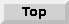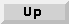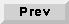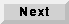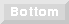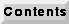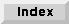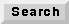## 8.4 An overview of BDF demos

The demos with icons shown in figure 8-2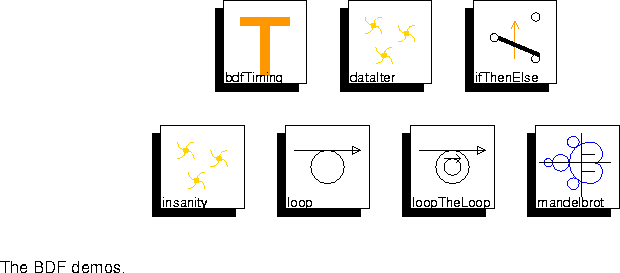illustrate Boolean-controlled dataflow principles. A useful way to understand these principles when running BDF demos is to display the schedule after a run. This can be done from pigi using the display-schedule command under the Exec menu. It must be done before the control panel is dismissed, because dismissing the control panel destroys the scheduler.

`bdfTiming` This demo is identical to the DDF timing demo, except that it uses BDF `Switch` and `Select` stars instead of DDF `Case` and `EndCase`. The static schedule has some simple if-then constructs to implement conditional firing.
`dataIter` This simple system, which does nothing interesting, is surprisingly difficult to schedule statically. It requires nesting an if-then within a do-while within a manifest iteration.
`ifThenElse` This simple system uses `Switch` and `Select` stars to construct an if-then-else.
`insanity` This peculiar system applies two functions, log and cosine, but the order of application is chosen at random. The BDF clustering algorithm fails to complete on this graph. If the allowDynamic parameter of the target is set to `YES`, then the scheduler will construct four SDF subschedules, which must then be invoked dynamically.
`loop` This system illustrates the classic dataflow mechanism for implementing data-dependent iteration (a do-while). A sequence of integers (a ramp) is the overall input. Each input value gets multiplied by 0.5 inside the loop until its magnitude is smaller than 0.5. Then that smaller result is sent to the output.
`loopTheLoop` This system is similar to the `loop` demo, except that a second do-while loop is nested within the first.
`mandelbrot` This system calculates the Mandelbrot set and uses Matlab to plot the output. Matlab must be installed on the local workstation to view the output of this demo, or Matlab must be available on a machine that is accessible via the Unix rsh command. See "Matlab stars" on page 5-26 for more information.